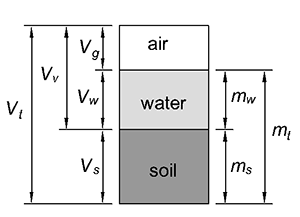# How to Calculate Bulk DensityBulk density, also called soil density, is the ratio of the total mass of a soil to the total volume.$\rho = \frac{m_t}{V_t}\newline\newline =\frac{m_w + m_s}{V_g + V_w + V_s}$
Where:
ρ = bulk density (kg/m3 or lb/ft3)
mt = Total mass (kg or lbs)
mw = Mass of water (kg or lbs)
ms = Mass of solids (kg or lbs)
Vt = Total Volume (m3 or ft3)
Vg = Volume of air (m3 or ft3)
Vw = Volume of water (m3 or ft3)
Vs = Volume of solids (m3 or ft3)

## Typical Bulk Densities

Soil Type Bulk Density
kg/m3 lb/ft3
Sand 1,600 – 2,000 100 – 125
Silt 1,200 – 1,350 75 – 84
Clay 1,100 – 1,400 69 – 87
Sandstone 2,150 – 2,650 134 – 165
Igneous Rocks 2,550 – 2,950 159 – 184

This site uses Akismet to reduce spam. Learn how your comment data is processed.